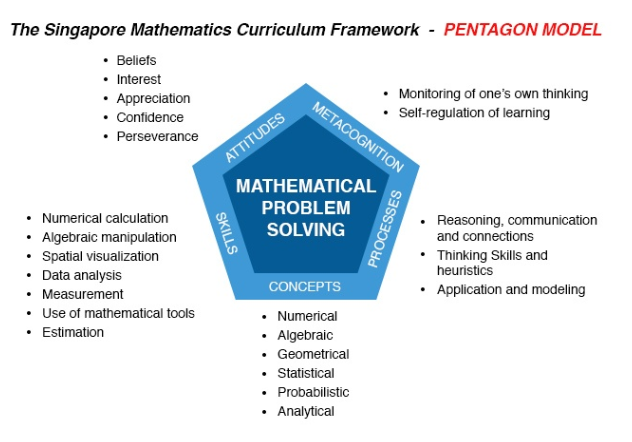# Singapore Math – Penang, Malaysia

Edunovice helps by providing Singapore Math tutoring to students in Penang, Malaysia. The math tutoring will be conducted in English and if students requires us to translate to Bahasa Melayu and Mandarin we would still be able to help with this request.

What is Singapore Math?
Mathematics problem solving are heuristics. The questions focuses on understanding, derivation of thinking box concept and visualization.  To understand more about the model of Singapore Math can be best explained by the Pentagon Model.Why should you learn Singapore math?

1. It is interesting because you learn to look at problems from different angle.
2. Some schools uses Singapore math to teach weak students and it is proven this improves their  Math marks
3. Better understanding on a specific problems and concepts